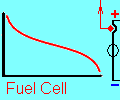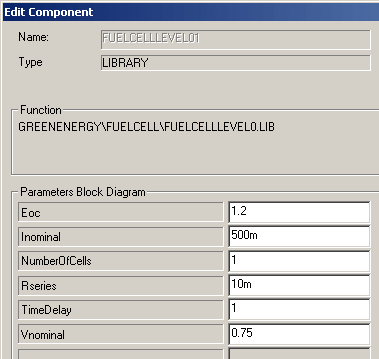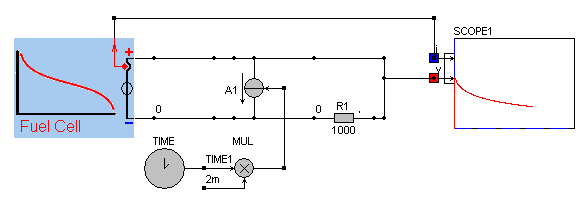• Introduction
• What is in this manual
• What is Caspoc
• User interface
• Introduction
• Starting
• Simulation
• Editing
• Viewing and printing
• Getting Started
• Basic editing
• Simulation in the time domain
• Basic User Interface Topics
• Editing
• Simulation
• Viewing
• Library
• Reports
• Project management
• Circuit and Block Diagram Components
• Introduction
• Cscript and user defined functions
• Component parameters
• Modeling Topics
• Introduction
• Power Electronics
• Semiconductors
• Electrical Machines
• Electrical drives
• Power Systems
• Mechanical Systems
• Thermal Systems
• Magnetic Circuits
• Green Energy
• Coupling to FEM
• Experimenter
• Analog hardware description language
• Embedded C code Export
• Coupling to Spice
• Small Signal Analysis
• Matlab coupling
• Tips and tricks
• Appendices

## Fuel Cell Level 0

Modeling a simple Fuel Cell.This simple fuel cell model is especially usefull when you quickly want to implement a fuel cell in your application without knowing the exact physical details of the fuel cell. Only the nominal voltage and current have to specified, as well as the open circuit voltage and the series resistance.The figure above shows the level 0 fuel cell model characteristic. Along the X-axis the current drawn from the fuel cell is displayed. The y-axis is the voltage per cell. By defining the number N of cells in series, the total voltage produced by the fuel cell is defined. The voltage of the fuel cell is depending on the current drawn form the fuel cell as indicated by the graph above.

The Fuel Cell library block is selected from the library at Components/Library/GreenEnergy/FuelCell

The Fuel Cell block has six parameters, which can be taken from the data sheet of hte fuel cel directly.Parameters of the level 0 Fuel Cell model:

• Eoc
Open circuit voltage is the maximum voltage of the fuel cell when no current is drawn from the cell.
• Inominal To specify the voltage-current characteristic, one voltage-current data point has to be defined. Inominal is the nominal current of one cell in the fuel cell. The choice of the optimal data point is described in the next section. Inominal is defined for one cell only.
• Vnominal To specify the voltage-current characteristic, one voltage-current data point has to be defined. Vnominal is the nominal current of one cell in the fuel cell. The choice of the optimal data point is described in the next section. Vnominal is defined for one cell only.
• Rseries The series resistance of the cell is defined here. This is a pure ohmic resistance and in this model not depending on temperature. The resistance is defined for one cell only.
• Number of Cells The NumberOfCells parameter specifies the number of cells in series. The cell open circuit voltage and the nominal voltage as well as the series resiance are multplied by this parameter.
• Time delay There is a time delay before the fuel cell responds to changes in the electrical load. This time delay is specified in seconds. For large integration step sizes dt, the time delay is internally limited by a minimum value of 10*dt.

The simulation below shows the characteristic of th level 0 fuel cell when loaded with a time varying load current. The fuel cell is connected to a load resistance and a controlled current. The load current controlled by multiplying the output form the block with 2m. The total simulation time is 1000 seconds and therefore the current increases form 0 to 2m*1000=2 amperes.
The time delay of the fuel cell is 1 second and the simulation time step dt is equal to 100ms. Therefore when starting the simulation, the maximum voltage of Eoc is nearly reached.The fuel cell electric terminals are on the right side of the block. The top connection is a measurement node and gives the value of the current drawn from the fuel cell. You can use the top connection for reading the value of the current that is flowing out of the fuel cell.